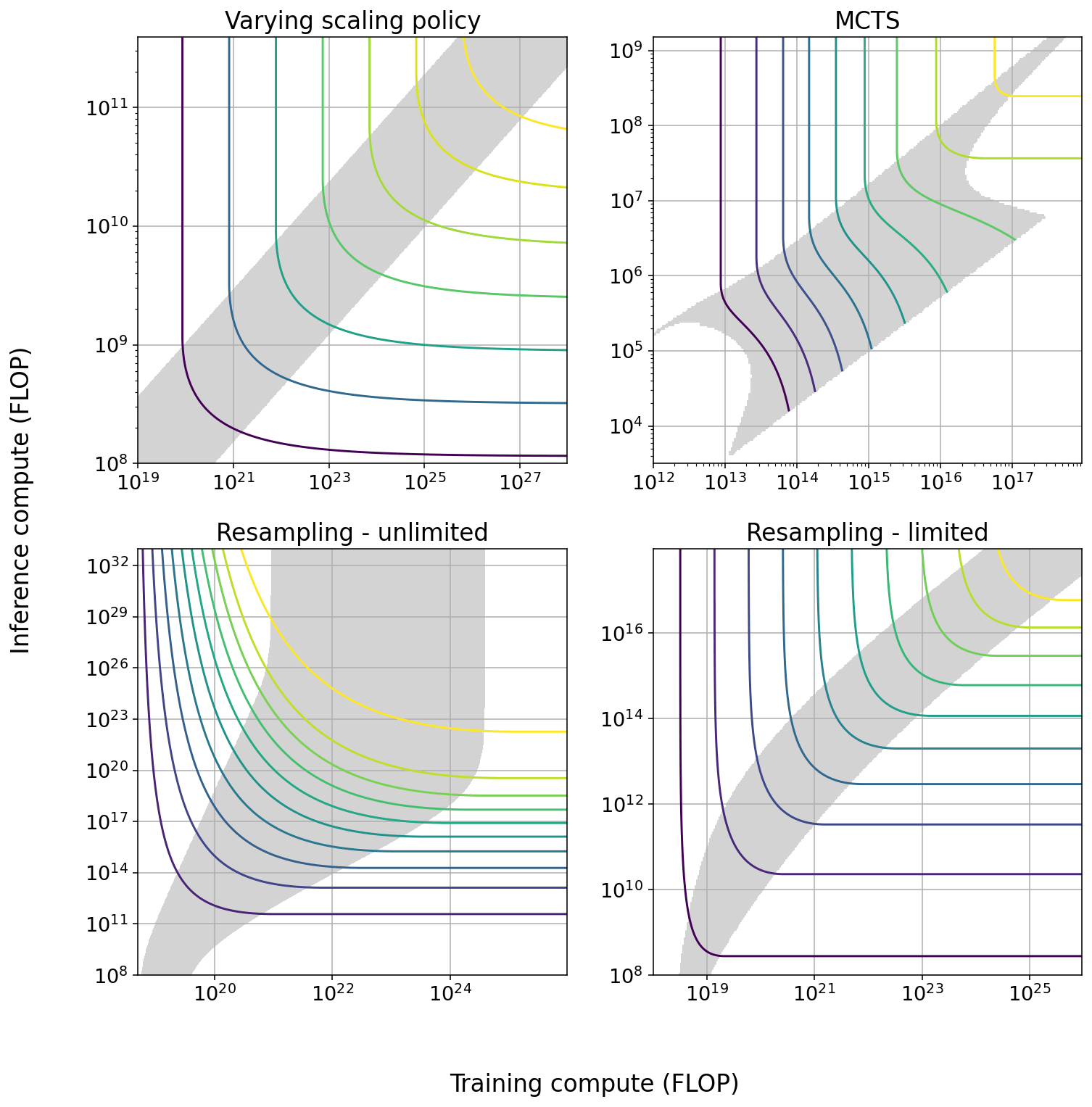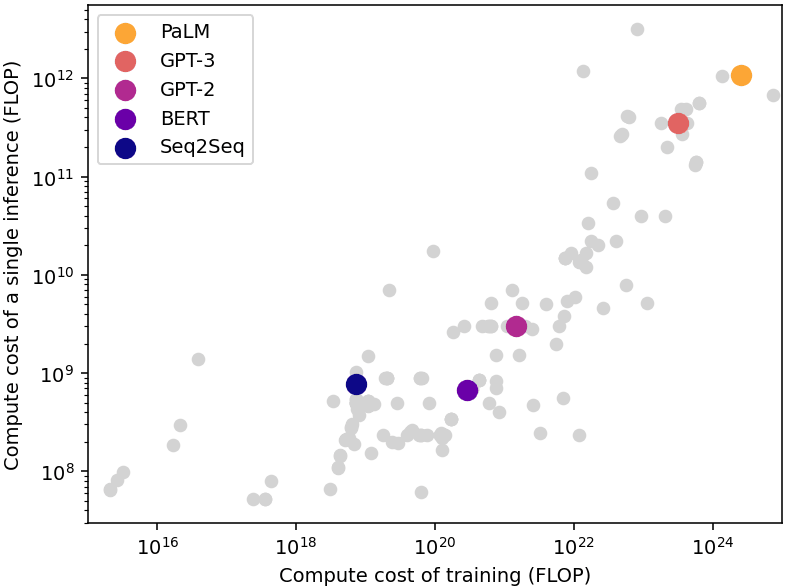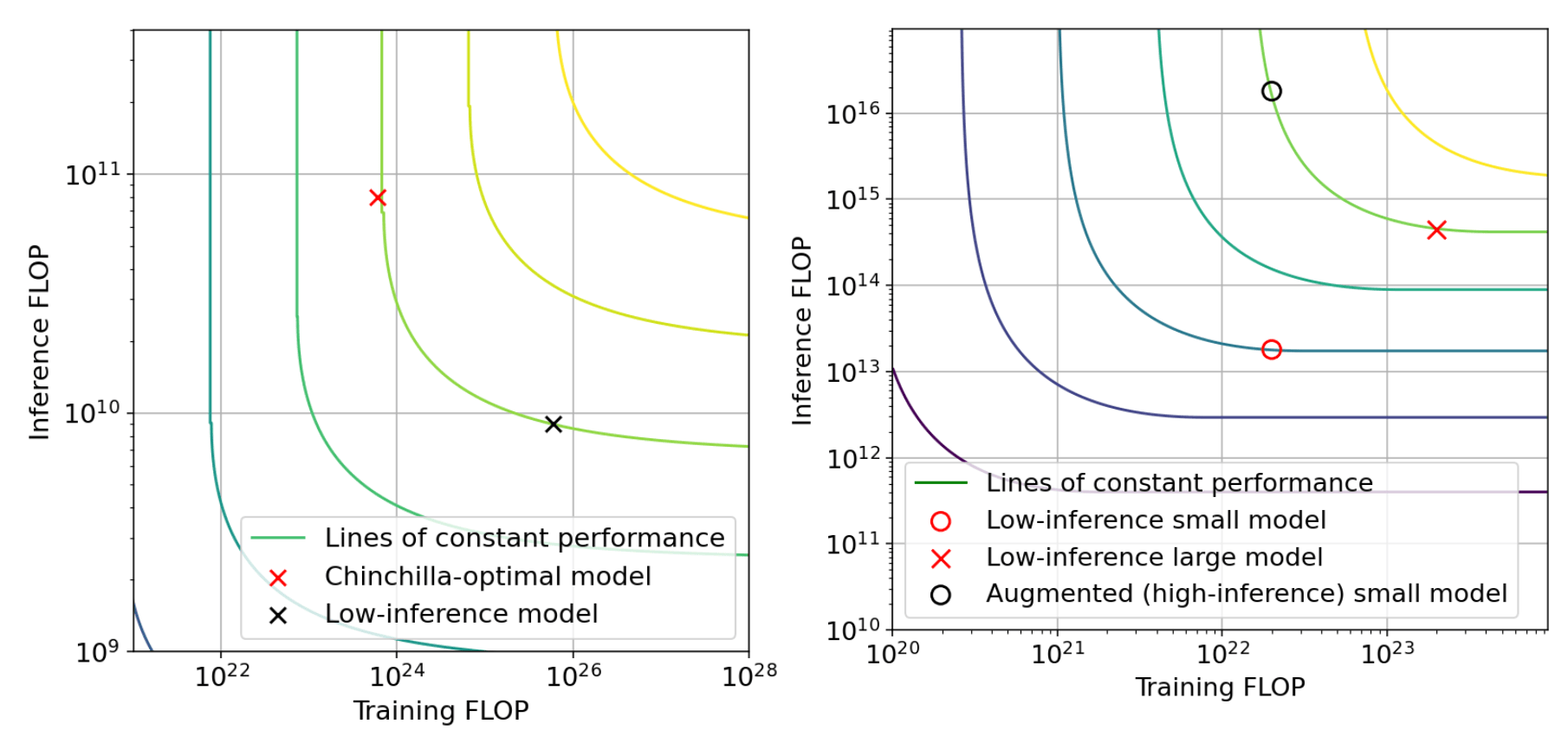# Trading off compute in training and inference (Overview)

8 min read31st Jul 20231 comment

# 30

Summary: Some techniques allow to increase the performance of Machine Learning models at the cost of more expensive inference, or reduce inference compute at the cost of lower performance. This possibility induces a tradeoff between spending more resources on training or on inference. We explore the characteristics of this tradeoff and outline some implications for AI governance.

# Key takeaways

In current Machine Learning systems, the performance of a system is closely related to how much compute is spent during the training process. However, it is also possible to augment the capabilities of a trained model at the cost of increasing compute usage during inference or reduce compute usage during inference at the cost of lower performance. For example, models can be pruned to reduce their inference cost, or instructed to reason via chains of thought, which increases their inference cost.

Based on evidence from five concrete techniques (model scaling, Monte Carlo Tree Search, pruning, resampling, and chain of thought), we expect that, relative to most current models (eg: GPT-4) it is possible to:

1. Increase the amount of compute per inference by 1-2 orders of magnitude (OOM), in exchange for saving ~1 OOM in training compute while maintaining performance. We expect this to be the case in most language tasks that don’t require specific factual knowledge or very concrete skills (eg: knowing how to rhyme words).
2. Increase the amount of compute per inference by 2-3 OOM, in exchange for saving ~2 OOM in training compute while maintaining performance. We expect this to be possible for tasks which have a component of sequential reasoning or can be decomposed into easier subtasks.
3. Increase the amount of compute per inference by 5-6 OOM in exchange for saving 3-4 OOM in training compute. We expect this to happen only for tasks in which solutions can be verified cheaply, and in which many attempts can be made in parallel at low cost. We have only observed this in the case of solving coding problems and proving statements in formal mathematics.
4. In the other direction, it is also possible to reduce compute per inference by at least ~1 OOM while maintaining performance, in exchange for increasing training compute by 1-2 OOM. We expect this to be the case in most tasks.

A key implication from this work is highlighting a tradeoff between model capabilities and scale of deployment. Since inference is the dominant cost for models deployed at scale, AI companies will apply some of these compute-saving techniques to minimize the inference costs of the models they offer to the public.

Meanwhile, these companies might be able to leverage additional inference compute to achieve better capabilities at a smaller scale, either for internal use or for a small number of external customers. Policy proposals which seek to control the advancement or proliferation of dangerous AI capabilities should take this possibility into account.Summary Figure: Tradeoff diagrams of the four techniques we studied in greatest depth. The solid curves indicate constant performance. The shaded region is the span of efficient exchange: the region in which it is possible to trade off the two types of compute at a marginal exchange rate better than 6 to 1. In some cases the size of the span increases with scale, in others it decreases with scale.

Summary Table: For each of the five techniques we studied, we display: the maximum size of the tradeoff in both training and inference compute; how the tradeoff behaves as models are scaled; and in which region of the tradeoff current models usually stand. ‘Minimal training compute’ indicates that current models are trained using as little training compute as possible given their performance.

# Introduction

The relationship between training compute and capabilities in Machine Learning systems is well known, and has been extensively studied through the lens of scaling laws. This relationship is responsible for the current concentration of advanced AI capabilities in companies with access to vast quantities of compute. It is also used for forecasting AI capabilities, and forms the technical foundation for some proposed policies designed to control the advancement and proliferation of frontier AI capabilities.

Less attention has been paid to the effect of inference compute on capabilities. While this effect is limited, we argue that it is significant enough to warrant consideration. There are multiple techniques that enable spending more compute during inference in exchange for improved capabilities. This possibility induces a tradeoff between spending more resources on training or spending more resources on inference.

The relationship between training and inference compute is complex: we must distinguish between the cost of running a single inference, which is a technical characteristic of the model, and the aggregate cost of all the inferences over the lifetime of a model, which additionally depends on the number of inferences run.

The cost of running a single inference is much smaller than the cost of the training process. A good rule of thumb is that the cost of an inference is close to the square root of the cost of training (see Figure A), albeit with significant variability. Meanwhile, the aggregated cost of inference over the lifetime of a model often greatly exceeds the cost of training, because the same model is used to perform a large number of inferences.Figure A: Compute required for training and running a single inference of multiple language models published since 2012. The single-inference compute is usually close to the square root of the training compute. Data from Epoch (2022).

## Individual techniques

We analyzed several techniques that make it possible to augment capabilities using more inference compute, or save inference compute while maintaining the same performance. The quantities of compute which can be traded off vary by technique and domain.

For example, using overtraining, it’s possible to achieve the same performance as Chinchilla, spending 2 OOMs of extra training compute in order to save 1 OOM of inference compute. Meanwhile, using resampling it’s possible to achieve the same performance as AlphaCode spending 1.5 OOM of additional inference compute in order to save 1 OOM of training compute. Both of these possibilities are illustrated in Figure B, which presents the tradeoff induced by these two concrete techniques.Figure B: Illustrative tradeoff examples. Left: tradeoff from overtraining in language modeling. The low-inference model (black) saves one order-of-magnitude (OOM) in inference by spending 2 additional OOMs in training, relative to a Chinchilla-optimal model. Right: tradeoff from resampling in n@k code generation. The high-inference model (red circle) saves 1 OOM in training compute by spending an additional 1.5 OOM in inference compute, relative to a non-augmented model (red x). Since augmentation can be done post-training, this means that a small model (red circle) can simulate the capability of a 1 OOM larger model, after being augmented with 3 OOM of additional inference compute.

Since there is significant variation between techniques and domains, we can’t give precise numbers for how much compute can be traded off in a general case. However, as a rule of thumb we expect that each technique makes it possible to save around 1 OOM of compute in either training or inference, in exchange for increasing the other factor by somewhat more than 1 OOM.

In addition, there is a class of tasks in which inference compute can be leveraged quite efficiently. In tasks where the solution generated by the AI can be cheaply verified and where failures are not costly (for example, writing a program that passes some automatic tests, or generating a formal proof of a mathematical statement) it is possible to generate a large number of solutions until a valid one is found.

For these tasks, it is possible to usefully spend an additional 6 orders of magnitude of compute during inference, and reduce training compute by 3 orders of magnitude. While we don’t expect many economically relevant tasks will have these characteristics, there might be a small number of important examples.

## Combining techniques

These techniques can be combined. We verified that at least in some cases, the effect of combining two techniques is as large as the combination of the individual effects. That is, if each technique allows 1 OOM of savings, using both techniques in combination allows 2 OOM of savings.

However, we believe this is a special case, and in general combining two techniques will produce less benefits than the sum of each individual technique. This is because each of these techniques can interfere with each other if they use the same mechanism of action. Therefore, we expect that only techniques which employ very different mechanisms of action will combine effectively.

After taking this into account, we believe using combinations of techniques can allow up to around 2-3 OOM of savings, because combining more than two techniques will usually be ineffective. Some of these techniques (in particular Chain of Thought and MCTS) seem to mostly produce benefits for tasks which have a compositional structure, like sequential reasoning. Other tasks might not see such large savings.

## Implications

The position of current models in these tradeoffs is crucial to evaluate their consequences. If current models are near the maximum inference compute for all techniques, it will be possible to save a lot of inference compute but no training compute at all, and vice versa.

The past generation of LLMs (GPT-3, PaLM, Chinchilla) would be placed close to a middle point in the combined tradeoff. This is because in each technique they are often in one of the extremes of the tradeoff, but they are in different extremes for different techniques. They use no pruning and no overtraining, so they are in the extreme of high inference compute for those techniques, but they also use no chain of thought or search, so they are in the extreme of low inference compute for those techniques.

While we know less about the latest-generation models (GPT-4, PaLM 2), and nothing about future models, we think they are likely stand closer to the low-inference end of the combined tradeoff, for the following reason:

The optimal balance between spending compute on training or inference depends on the number of inferences that the model is expected to perform. In applications which are expected to require a large number of inferences (eg: deploying a system commercially), there is an economic incentive to minimize inference costs. In the opposite case, for applications which are expected to only require a small number of inferences (eg: evaluating the capabilities of a model), there is no such incentive since training is the dominant cost.

As a consequence, it seems likely that models deployed at scale will be closer to the low end of inference compute. Meanwhile, there will be substantially more capable versions of those models that use more inference compute and therefore won’t be available at scale.

# Conclusions

Spending additional compute during inference can have significant effects on performance. This is particularly true for models deployed at scale by AI companies or open source collectives, which have an incentive to produce models that are optimized for running cheaply during inference.

In some cases, it might be possible to achieve the same performance as a model trained using 2 OOM more compute, by spending additional compute during inference. This is approximately the difference between successive generations of GPT models (eg: GPT-3 and GPT-4), without taking into account algorithmic progress. Therefore it should be possible to simulate the capabilities of a model larger than any in existence, using current frontier models like GPT-4, at least for some tasks. Even larger improvements might be possible for tasks where automatic verification is possible.

This has several consequences for AI governance. While these amplified models will not be available at scale, some other actors might be able to leverage them effectively. For example:

1. Model evaluations and safety research can use these techniques to anticipate the capabilities that will become available at scale in the future.
2. AI progress might be faster than expected in some applications, at a limited scale due to higher inference costs. For example, AI companies might be able to use augmented models to speed up their own AI research.
1. ^

Since the techniques we have investigated that make this possible, overtraining and pruning, are extremely general. Other techniques such as quantization also seem very general.

2. ^

Patterson et al. (2022) find that the aggregate cost of inference at Google data centers in three weeks of 2019, 2020 and 2021 accounts for 60% of the total ML compute expenditure. In addition, the fact that as of july 2023 GPT-4 is only available with a rate limit in ChatGPT, even for paying customers, suggests that inference is currently a bottleneck for AI companies.

3. ^

This is already the case, with quantization being commonly used in open-source models, and speculatively also in closed-source models.

4. ^

Span in inference compute increases by roughly 0.08 OOM for each OOM increase in training compute. The efficiency of the tradeoff (the ratio of inference compute reduction to training compute increase) increases by 0.5 for each OOM increase in training compute.

5. ^

Note that we are extrapolating over many orders of magnitude and we should treat this result with skepticism.

6. ^

Span in both training and inference compute increases by 0.75 OOM for each OOM increase in training compute.

7. ^

We could not determine this quantity for chain of thought-style techniques, since they are usually binary: either they are employed or not, with no possibility of continuous variation in the intensity of usage.

8. ^

See, for example, Owen (2023).

9. ^

Some examples are the proposed moratorium on training runs beyond a certain size, and the proposal to require a license to train large models.

10. ^

For example, for GPT-3, the cost of training was 3e23 FLOP, whereas the cost of a single inference is 3e11. So the cost of training is equivalent to performing 1e12 inferences.

11. ^

Patterson et al. (2022) find that the aggregate cost of inference at Google data centers in three weeks of 2019, 2020 and 2021 accounts for 60% of the total ML compute expenditure.

12. ^

We expect that only tasks that involve achieving a concrete goal in a formal system will admit cheap verification. One example is playing video games, but it does not have significant economic impact. Hacking and chip design are more relevant possibilities.

13. ^

For example, both overtraining and pruning rely on reducing the number of parameters in the model, so they likely can’t be combined effectively. Meanwhile, overtraining and MCTS can be effectively combined.

14. ^

Assuming the task at hand does not admit cheap verification. If it does, the combined tradeoff might span 6 OOM or more.

15. ^

For example, if we assume that 300M users are querying a model an average of once per day for a year, and each query consumes 1000 tokens, the number of forward passes in a year will be 300e6 * 365 * 1000 = 110 trillion. Meanwhile, training a model the size of GPT-3 compute-optimally requires 3.5 trillion backward passes, each of which is 3x as expensive as a forward pass. So the cost of training is only 1/10rd the cost of inference over a year. Therefore, it might make sense to overtrain a smaller model to minimize the total cost.

# 30

New Comment
1 comment, sorted by Click to highlight new comments since: